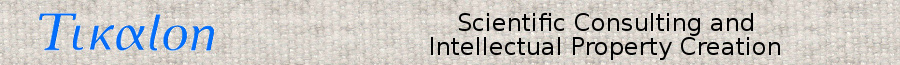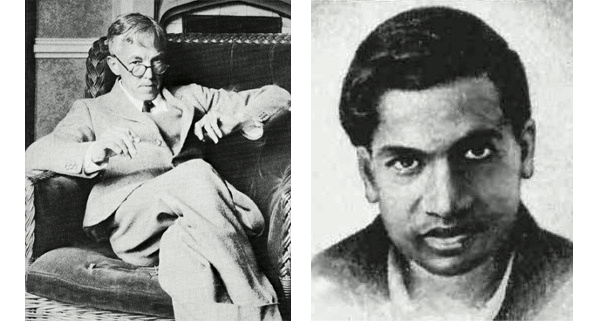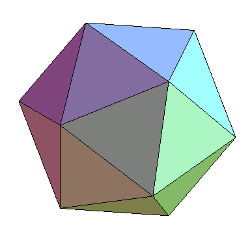### Special Numbers

September 21, 2011

Heraclitus, who is famous for his statement that you can't step in the same river twice, knew that there is a level at which everything is unique. Those identical M&M candies can be distinguished from each other with a sensitive enough pan balance. The trick to this game is to look far enough to find whatever makes an item special.

Numbers such as pi and e are clearly special, but what makes a natural number like 1729 special? The mathematician, G.H. Hardy once visited the Indian mathematician Srinivasa Ramanujan in the hospital, and he told this story.
"I remember once going to see him when he was ill at Putney. I had ridden in taxi cab number 1729 and remarked that the number seemed to me rather a dull one, and that I hoped it was not an unfavorable omen. "No," he replied, "it is a very interesting number; it is the smallest number expressible as the sum of two cubes in two different ways."G.H. Hardy (left), circa 1927, from his book, "A Mathematician's Apology," and an undated photograph of Srinivasa Ramanujan (right). (Via Wikimedia Commons: (Left Image), (Right Image).

The number, 1729, is now known as the second taxicab number. The different ways are 13 + 123 and 93 + 103. That Ramanujan was in poor enough health to be in a hospital was not unexpected. The combination of his trying to eat vegetarian in early twentieth century England and his frenetic work habits likely contributed to his early demise at age 32. We can only guess what he may have discovered in number theory if he had lived longer.

Erich Friedman, an Associate Professor of Mathematics at Stetson University has memorized the first fifty digits of pi, compared to my paltry eleven. He also has an extensive website devoted to the things that make natural numbers special. Some of the reasons are quite imaginative. Here are a few examples.
18 is the only positive number that is twice the sum of its digits.

24 is the largest number divisible by all numbers less than its square root.

26 is the only positive number to be directly between a square and a cube.

31 is a Mersenne prime.

38 is the last Roman numeral when written lexicographically; i.e., alphabetically (XXXVIII).59 is the number of stellations of an icosahedron.

77 is the largest number that cannot be written as a sum of distinct numbers whose
reciprocals sum to 1.

100 is the smallest square which is also the sum of 4 consecutive cubes (1+8+27+64).

125 is the only number known that contains all its proper divisors as proper substrings.

151 is a
palindromic prime.

162 is the smallest number that can be written as the sum of of 4 positive squares in 9 ways.

171 has the same number of digits in Roman numerals as its cube. (CLXXI and |L|CCXI, where |L| is counted as a single digit)

199 is the eleventh Lucas number.

206 is the smallest number whose English name contains all five vowels exactly once (Two hundred and six). If you allow the "sometimes y" criterion, it would be two hundred and sixty.

337 is the number of different resistances that can be created in a circuit of 8 equal resistors. (Electrical engineering and computer science professors, note: This would be a good homework problem.)

380 is the number of necklaces possible with 13 beads, each being one of 2 colors.

394 is a Schröder number.

418 has the property that the sum of its prime factors is equal to the product of its digits.

428 has the property that its square, 183184, is the concatenation of two consecutive numbers.

512 is the cube of the sum of its digits.

855 is the smallest number which is the sum of 5 consecutive squares or 2 consecutive cubes.

1964 is the number of legal knight moves in chess.

1998 is the largest number that is the sum of its digits and the cube of its digits.

2034 is the number of self-avoiding walks of length 9.

5913 = 1! + 2! + 3! + 4! + 5! + 6! + 7!

5914 = 0! + 1! + 2! + 3! + 4! + 5! + 6! + 7!

6211 is a Cuban prime; that is, it's a member of Sloane's Integer Sequence A002407.

9996 has a square, 99920016, formed by inserting a block of digits inside itself.

### References:

Linked Keywords: Heraclitus; M&M candies; pi; e; natural number; 1729; mathematician; G. H. Hardy; Indian; Srinivasa Ramanujan; A Mathematician's Apology; Wikimedia Commons; taxicab number; vegetarian; twentieth century; England; number theory; Erich Friedman; mathematics; Stetson University; Mersenne prime; Roman numeral; lexicography; stellation; icosahedron; reciprocal; palindromic prime; Lucas number; electrical resistance; resistor; electrical engineering; computer science; homework; Schröder number; prime factor; concatenation; knight; chess; self-avoiding walk; Cuban prime; Sloane's Integer Sequence A002407.